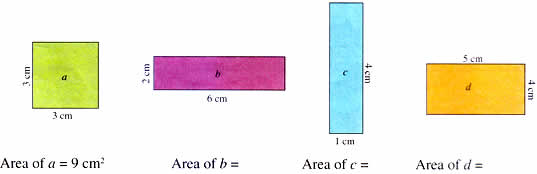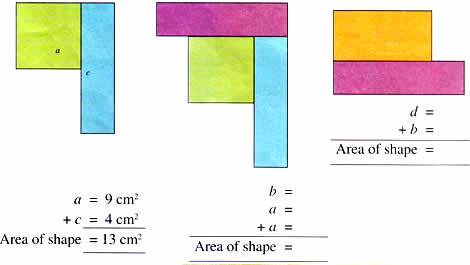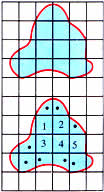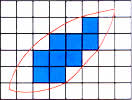Thursday , July 7 2022# NCERT 5th Class (CBSE) Mathematics: Area And Volume

## FINDING THE AREA OF OTHER SHAPES

### Area of Combined Shapes

This window has a pattern made out of stained glass.

These are pieces of stained glass used to make colourful windows. Find the areas of each.These pieces of stained glass have been combined to make some basic patterns. Find the area of these combined shapes by adding the areas of the shapes that they are made up of.### Area of an Irregular Figure

So far you have been estimating the area of a irregular figure by counting all the full squares and combine partial squares to make whole squares (refer page 1 of this chapter).

There are two other ways to estimate the are of an irregular shape.

Method 1

This is the map of a lake. Find the area of the lake on the map. Take each square to be 1 sq. km.Step 1: Count the whole squares = 5

Step 2: Count the parts that are half of more (marked with a • ) = 8

Do not count the parts that are less than half.

Step 3: Add the 2 figures

8 + 5 = 13.

The area of this shape is about 13 sq. km.

Method 2Step 1: Count all the whole squares = 9

Step 2: Count all the squares – both whole and part = 25

Step 3: Add the numbers obtained in steps 1 and 2 = 9 + 25 = 34.

Step 4: Divide that total by 2 to find the approximate area of the irregular shape = 34 ÷ 2 = 17

Area = about 17 sq. units

## चुनौती हिमालय की 5th NCERT CBSE Hindi Rimjhim Ch 18

चुनौती हिमालय की 5th Class NCERT CBSE Hindi Book Rimjhim Chapter 18 प्रश्न: लद्दाख जम्मू-कश्मीर राज्य में …